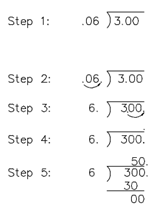## Dividing Decimals Assignment Help

Assignment Help: >> Decimals - Dividing Decimals

Dividing Decimals:

While  solving  problems  including  division  of  decimals,  the  subsequent  procedure  should  be applied.

Step 1:  Write out the division problem.

Step 2:  In the divisor Move the decimal to the right.

Step 3:  Move the decimal in the dividend the same number of places to the right. Add an zeros after the decimal within the dividend if necessary.

Step 4:  Put the decimal point in the quotient directly above the decimal in the dividend.

Step 5:  Divide the numbers. Example:

3.00  ÷  0.06

Solution: